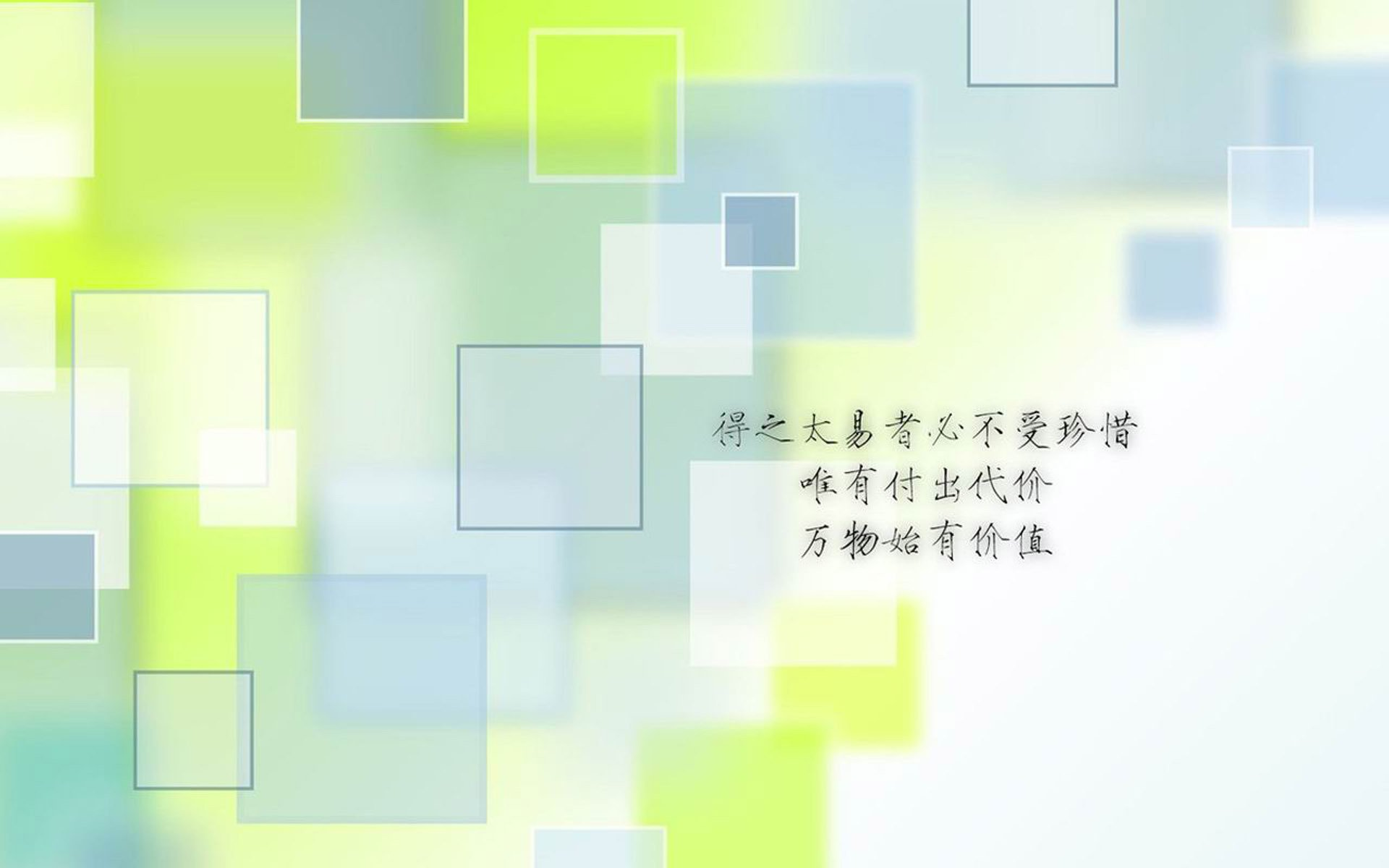# 空间彩色留言,QQ空间怎样做个性的彩色留言？QQ空间个性留言代码 /qzone/gexing/

QQ空间彩色留言代码 /qzone/caise/

QQ空间非主流留言代码 /qzone/fzl/

QQ空间互踩留言代码 /qzone/hucai/

QQ空间霸屏留言代码 /qzone/baping/

## 推荐几个好的空间彩色留言代码

[M][ftf=Webdings][fts=6][ftc=EE1000]Y[/ft][/ft][/ft]

[ftf=Webdings][fts=6][ftc=F7941D]Y[/ft][/ft][/ft] [em]e41[/em] [em]e41[/em] [ftf=Webdings][fts=6][ftc=ED008C]Y[/ft][/ft][/ft][/M]

[M][ftc=FFF100]親[/ft] [ftc=00AEEF]愛[/ft] [ftc=8FC63D]啲[/ft] [/M]

[M][ftc=F16522]∨[/ft] [ftc=00A99E]硪[/ft] : ˉ[ffg,ED008C,FFFFFF]最 喜 歡 祢 叫 咱 寶 貝[/ft] 、[/M]

[M][ftc=FFF100]√[/ft] [ftc=00AEEF]√[/ft] [ftc=ED008C]√[/ft] [ftc=F7941D]√[/ft] [ftc=37B400]√[/ft] [ftc=EE1D24]√[/ft] [ftc=00A99E]√[/ft] [ftc=EF6EA8]√[/ft] [ftc=FDC68C]√[/ft] [ftc=FFF100]√[/ft] [ftc=7CA6D8]√[/ft] [ftc=81CA9D]√[/ft] [ftc=ACD372]√[/ft][/M]

[M][ftc=F7941D]春 天 的 时候[/ft] 、[ftc=FFF100]我[/ft] [ftc=00AEEF]把[/ft] [ftc=ED008C]宝[/ft] [ftc=8FC63D]贝[/ft] [ftc=00BFF3]种[/ft] [ftc=F16D7E]在[/ft] ≮[B][ftc=EE1000]地 里[/ft][/B]≯ [/M]

[M][ftc=F16522]到[/ft] [ftc=00A99E]了[/ft] [U][ftc=8FC63D]秋 天[/ft][/U] 、[ftc=7CA6D8]<b>就 会 结 出 许 多 个 宝 贝</b> [/ft]、[/M]

[M][ftc=FFF100]会[/ft] [ftc=00A99E]结[/ft] [ftc=ED008C]出[/ft] [ftc=8FC63D]各[/ft] [ftc=FBAF00]式[/ft] [ftc=00A650]各[/ft] [ftc=F16D7E]样[/ft] [ftc=8FC63D]的[/ft] [ftc=ED008C]宝 贝[/ft] 、 到 时 候 【[B][ftc=EE1000]丢 一 个[/ft][/B]】 也 没 事 、[/M]

[M][ftc=00AEEF]因 为 我 还 有[/ft] ［[B][ftc=FFF100]忒 多 捏[/ft][/B]］ 、[ftc=37B400]喂[/ft]、[ftc=F7941D]不 许 一 个 人 偷 偷 的 拿 走[/ft] ， [/M]

[M][ftc=EE1000]<b>[B]等 宝 贝 结 出 来 我 可 以 送 给 你 一 个 的</b>[/B][/ft] 、[/M]